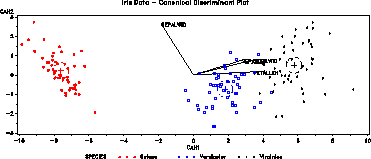canplot Canonical discriminant structure plot canplot

# SAS Macro Programs: canplot

\$Version: 1.6-5 (22 May 2007)
Michael Friendly
York University

## The canplot macro (get canplot.sas)

### Canonical discriminant structure plot

The canplot macro constructs a canonical discriminant structure plot. The plot shows class means on the two largest canonical variables, confidence circles for those means, and variable vectors showing the correlations of variables with the canonical variates.

### Method

Discriminant scores and coefficients are extracted from PROC CANDISC and plotted.

Other designs may be handled either by (a) coding factor combinations 'interactively', so, e.g., the combinations of A*B are represented by a GROUP variable, or (b) by applying the method to adjusted response vectors (residuals) with some other predictor (class or continuous) partialled out. The latter method is equivalent to analysis of the residuals from an initial PROC GLM step, with the effects to be controlled or adjusted for as predictors.

e.g., to examine Treatment, controlling for Block and Sex,

```   proc glm data=..;
model Y1-Y5 = block sex;
output out=resids
r=E1-E5;
%canplot(data=resids, var=E1-E5, class=Treat, ... );
```

## Usage

canplot is a macro program. Values must be supplied for the CLASS= and VAR= parameters.

The interpretation of the angles betweeen variable vectors relies on the units for the horizontal and vertical axes being made equal (so that 1 data unit measures the same length on both axes. The axes should be equated either by using the GOPTIONS HSIZE= VSIZE= options, or using the macro HAXIS= and VAXIS= parameters and AXIS statements which specify the LENGTH= value for both axes.

The current version now uses the equate macro if the HAXIS= and VAXIS= arguments are not supplied.

The arguments may be listed within parentheses in any order, separated by commas. For example:

```   %canplot(data=inputdataset, var=predictors, class=groupvariable..., )
```

### Parameters

The current version now provides many more parameters; see the program header.
DATA=_LAST_
The name of the input dataset. If not specified, the most recently created dataset is used.
CLASS=
The name of one class (group) variable
VAR=
List of classification (predictor) variables
SCALE=AUTO
Scale factor for variable vectors in plot. The variable vectors are multiplied by the SCALE= value, which should be specified (perhaps by trial and error) to make the vectors and observations fill the same plot region. If SCALE=AUTO or SCALE=0, the program estimates a scale factor from the ratio of the maximum distance to the origin in the observations relative to the variables.
CONF=.95
Confidence probability for canonical means
OUT=_DSCORE_
The name of the output data set containing discriminant scores
ANNO=_DANNO_
Output data set containing annotations
PLOT=YES
YES to produce the plot, or NO to suppress the plot
HAXIS=
The name of an optional AXIS statement for the horizontal axis. The HAXIS= and VAXIS= arguments may be used to equate the axes in the plot so that the units are the same on the horizontal and vertical axes.
VAXIS=
The name of an optional AXIS statement for the vertical axis.
NAME=CANPLOT
name for graphic catalog entry
COLORS=RED GREEN BLUE BLACK PURPLE YELLOW BROWN ORANGE
List of colors to be used for groups
SYMBOLS=+ SQUARE STAR - PLUS : \$ =
List of symbols to be used for the various groups (levels of the CLASS= variable).

### Example

The example below plots the canonical structure of the Iris data. The graphic options HSIZE and VSIZE are used to scale the plot so that 1 unit on the horizontal and vertical axes are approximately the same size.
```goptions vsize=3.2 in hsize=7.5in  htext=2;
%include data(iris);

title h=2.5 'Iris Data - Canonical Discriminant Plot';
axis1 order=(-10 to 10 by 2);
axis2;
legend1 value=(h=2);
%canplot(
data=iris, class=species,
var=sepallen sepalwid petallen petalwid,
haxis=axis1, vaxis=axis2, legend=legend1, hsym=1.5,
colors=red blue black, scale=3.5);
```### See also

boxglm Power transformations by Box-Cox method for GLM
equate Creates AXIS statements for a GPLOT with equated axes
hemat HE plots for all pairs of response variables
heplot Plot Hypothesis and Error matrices for a bivariate MANOVA effect
meanplot Plot means for factorial designs
outlier Robust multivariate outlier detection